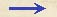Discorsi Propositions 3/09-th-06-lemma Discorsi Proposition3/09-th-06-lemmaLEMMA PRO SEQUENTI. LEMMA FOR THE FOLLOWING PROPOSITIONSi recta linea secta fuerit utcumque, quadrata mediarum inter totam et partes aequalia sunt quadrato totius. If a straight line be cut at any point whatever and mean proportionals between this line and each of its parts be taken, the sum of the squares of these mean proportionals is equal to the square of the entire line. Secta sit ab utcunque in c: dico, quadrata linearum mediarum inter totam ab et partes ac, cb, simul sumpta, aequalia esse quadrato totius ab. Id autem constat, descripto semicirculo super tota ba, et {299} ex c erecta perpendiculari cd, iunctisque da, db. Est enim da media inter ba, ac, estque db media inter ab, bc; suntque quadrata linearum da, db, simul sumpta, aequalia quadrato totius ab, recto existente angulo adb in semicirculo. Ergo patet propositum. Let the line ab be cut at c. Then I say that the square of the mean proportional between ab and ac plus the square of the mean proportional between ab and cb is equal to the square of the whole line ab. This is evident as soon as we describe a semicircle upon the entire line ab, erect a perpendicular cd at c, and draw da and db. For da is a mean proportional between ab and ac while {299} db is a mean proportional between ab and bc: and since the angle adb, inscribed in a semicircle, is a right angle the sum of the squares of the lines da and db is equal to the square of the entire line ab. Hence follows the proposition.Discorsi Propositions 3/09-th-06-lemma Discorsi Proposition3/09-th-06-lemma